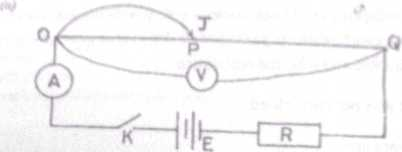Physics Paper 1A, May/June. 2012
 Questions: 1 2 3 Main
Weakness/Remedies
Strength

Question 3A

Question 3You are provided with a potentiometer, an ammeter, a voltmeter, standard resistor and other necessary apparatus. Using the circuit diagram above as a guide, carry out the following instructions.

1. Set up a circuit as illustrated in the diagram above.
2. Close the key, K.
3. Read and record the ammeter reading l0 and the voltmeter      reading V0 when     jockey J is not making contact with the potentiometer wire OQ
4. Using J, make a contact with the potentiometer wire OQ at a point P such that OP = 10 cm
1. Read and record the current I and the corresponding value of the voltage V
2. Repeat the procedure for other values of OP = 20 cm, 30cm, 40 cm, 50 cm, and 60cm.
2. Plot a graph with V on the vertical axis and I on the horizontal axis, starting both axes from the origin (0,0).
3. Determine the slope, s, of the graph.
4. Determine the value of V when I = 0.
5. State two precautions taken to obtain accurate      results.

(b) (i) State two advantages of a lead-acid accumulator over a dry Leclanche cell.
(ii) A cell of emf 2V and internal resistance of IQ passes current through an external load
of 9Q. Calculate the potential drop across the cell.

_____________________________________________________________________________________________________
observation

This is another popular question among the candidates and it was well attempted by majority of the responding candidates

However, some candidates missed valuable marks on the observation because their values did not tally with the required trend expected of them. Few other candidates recorded the values of OP without decimal place while some did not begin the graph from the origin (0,0), so they could not determine V when I 0 correctly.
In part (b) most candidates could not compare very well lead aid accumulator with dry lechlanche cell. Most candidates only compared the rechargeable aspect while they left out polarization, local action and internal resistance.
The numerical part was poorly tackled.
(t)          I0  read and recorded to at at least 1 d.p in ampere
(ii)        V„ read and recorded to at least 1 d.p in volts
(iii)       Five    values of I correctly read and recorded
to at least 1 d.p in ampere and in trend (Trend: As OP increases, I increases)
(iv)      Six   values of OP correctly recorded to at least to  1 d.p in cm
(v)      Six values of V read and recorded to at least I d.p in volts and in trend
Trend: As OP increases V decreases
(vi)      Composite table showing at least I, OP and V

Candidates are also expected to;
(i) Plot six points on graph
(ii) Distinguish between the axes
(iii) Choose reasonable scales
(iv) Draw line of best fit
(v) Determine the slope of the graph
(vi) Deduce the value of V when I = 0
(vii) State any two of the following precautions in acceptable language.
-- Open key when not taken readings
-- Avoided parallax error in readings ammeter/voltmeter
-- Zero error was noted and corrected for on ammeter/voltmeter
-- Clean and tight terminals Jockey not slide/drag on potentiometer wire